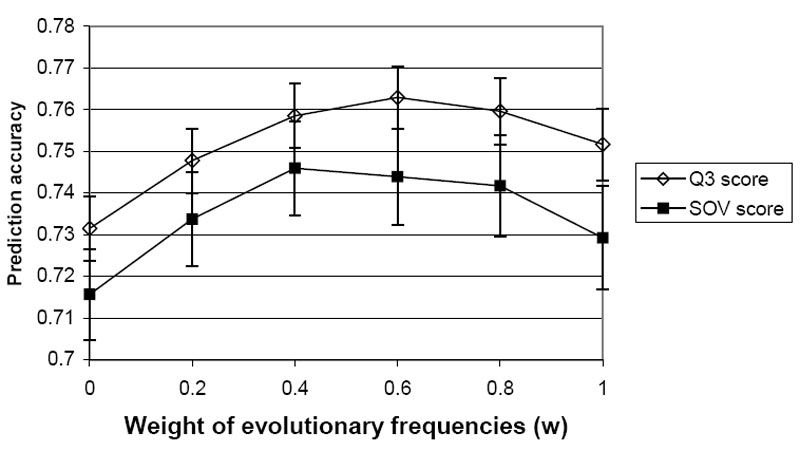Local structure prediction by combining evolutionary and structural information. Amino acid frequencies are estimated using two different methods: (1). from multiple sequence alignment (evolutionary frequencies) and (2). from local structure propensities derived by statistical analysis of a set of protein structures (structure frequencies). An optimal linear combination of these two types of frequencies:
F(combined) = w · F(evolutionary) + (1-w) · F(structure)
can give better local structure prediction accuracies than using either frequencies alone, as reflected by the Q3 and SOV scores of secondary structure prediction. In this diagram, when weight (w) is zero, only structural frequencies are used. when weight is 1, only evolutionary frequencies are used. The optimal weight is about 0.6.